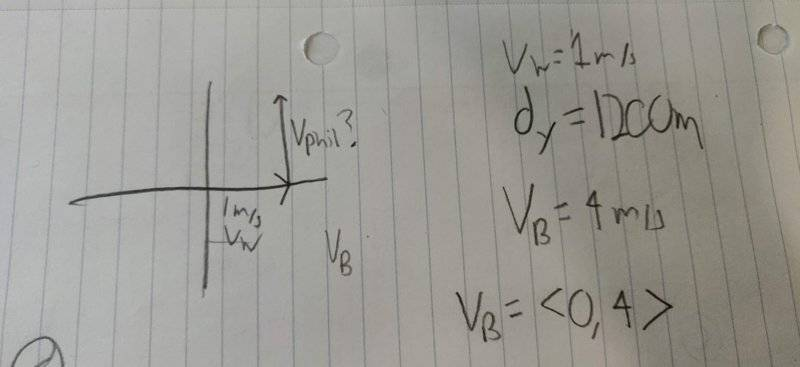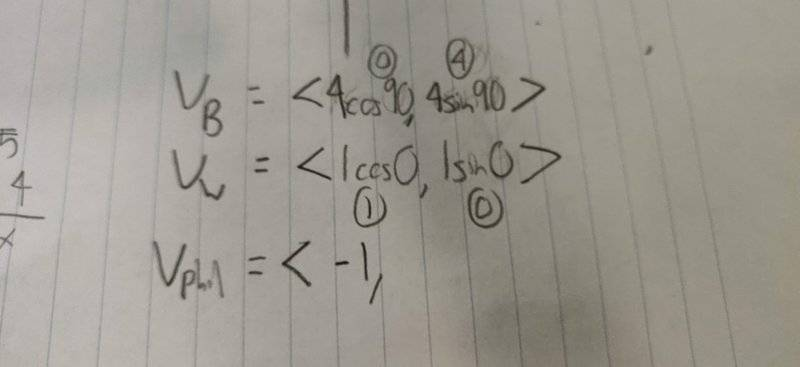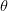# Adding Vectors to Find Velocity

Problem: Dr. L and his cat Kepler are coming home from fishing. They ended their trip on the south bank of a river, directly across a 1200 m wide river, from where Caroline was going to pick them up on the north bank. They are in identical boats that can travel at 4 m/s (Vb). The river is normally 'lazy' and flows with negligible velocity. On a calm day, if they pointed the boats north, they would cross the river in exactly 300 s. However, today the river is flowing due east with a constant velocity (Vw) of 1 m/s relative to the ground. Kepler, keeps the boat pointed due north for the entire trip.

*Phil notices what happened to Kepler when she fails to deal with the flow. He takes the water into account so that the path of his boat relative to the ground is due north. How long does it take Phil to cross the rover?
-------------------
Hello all, so my problem is with part 3, where it asks how long it takes for Phil to cross the river when he takes into account the current of the river (1 m/s East). I think what needs to be done is a calculation for Phil's velocity but I'm not sure how to go about this. So the problem says the boat can go 4 m/s (Vb), and I'm not sure how to configure the vectors to find the resultant velocity. I keep getting a setup like that I did in part one to find the cats velocity when it doesn't take into account the flow of water.

I have attached images of the problem, my solution for part 1, and my attempt at part 3.

Any help would be greatly appreciated!

P.S. I would love some tips on how to organize and label variables as I struggle with that.

#### Attachments

PeroK
Homework Helper
Gold Member
2020 Award
My tip is this. Imagine the boat and the water moving separately every 1s.

Draw where the boat moves in 1s, relative to the water and the bank, as though the water is not moving. Then, move the water with the boat relative to the bank.
Repeat.

From this you should see what's happening.

My tip is this. Imagine the boat and the water moving separately every 1s.

Draw where the boat moves in 1s, relative to the water and the bank, as though the water is not moving. Then, move the water with the boat relative to the bank.
Repeat.

From this you should see what's happening.
That sounds like how to solve part 1 I think? Part 1 the cat just goes forward and doesn't account for the current. But when Phil goes the problem says he "takes the water into account so that the path of his boat relative to the ground is due north", which I'm not exactly sure what that means. So are there three vectors I need to work with (Velocity of phil, the water, and the boat)? And how do I set them up, because when I set them up I keep getting a similar setup with part 1, where the boat is vertical velocity and the current is horizontal velocity. I think I am confused on the wording of whats happening#### Attachments

PeroK
Homework Helper
Gold Member
2020 Award
Try something other than a velocity at right angles to the water/current.

Try something other than a velocity at right angles to the water/current.
But why would they be anything other than right angles? From my understanding the water is the velocity in the x direction and the boats velocity is in the y direction. For finding the resultant velocity into account it was easy to just find the resultant vector of those two vectors. However not Phil "counteracts" that x velocity, which would make the x velocity 0, and leaving only the 4 m/s but I know that's not his final velocity. I also tried to subtract the boats velocity by the waters velocity but that brings me to the same 4.12 m/s answer from part 1.

Is this possible to do by breaking it down into components, I have the components of the velocity of water and the boat and what I would think phils velocity in the x direction would need to be to counter act the velocity of water. Or is this a dead end?
Thanks so much#### Attachments

PeroK
Homework Helper
Gold Member
2020 Award
But why would they be anything other than right angles? From my understanding the water is the velocity in the x direction and the boats velocity is in the y direction. For finding the resultant velocity into account it was easy to just find the resultant vector of those two vectors. However not Phil "counteracts" that x velocity, which would make the x velocity 0, and leaving only the 4 m/s but I know that's not his final velocity. I also tried to subtract the boats velocity by the waters velocity but that brings me to the same 4.12 m/s answer from part 1.

Phil can row his boat in whatever direction he likes: upstream, downstream, crossstream and anything in between.

Imagine you were at the swimming pool. You don't physically have to swim at right angles to the side of the pool.

•Charge
Chestermiller
Mentor
Let ##v_x## represent the x component of Phil's velocity relative to the flowing water, and ##v_y## represent the y component of Phil's velocity relative to the flowing water. If Phil's actual velocity relative to the ground is zero (his boat is moving due North), what is the value of ##v_x##? Since the magnitude of his total velocity relative to the flowing water is 4 m/s, what is the value of ##v_y##? Is this also equal to his velocity in the y direction relative to the ground?

Let ##v_x## represent the x component of Phil's velocity relative to the flowing water, and ##v_y## represent the y component of Phil's velocity relative to the flowing water. If Phil's actual velocity relative to the ground is zero (his boat is moving due North), what is the value of ##v_x##? Since the magnitude of his total velocity relative to the flowing water is 4 m/s, what is the value of ##v_y##? Is this also equal to his velocity in the y direction relative to the ground?

vx would be -1, I'm thinking because that would cancel out with the velocity of the current. I would also think that the x component of his velocity relative to the water and the ground would be the same because there is no velocity in the y direction from the water. The thing that confuses me, that if vx does equal -1, wouldn't that just cancel out the velocity from the water?
Thanks!

Chestermiller
Mentor
vx would be -1, I'm thinking because that would cancel out with the velocity of the current. I would also think that the x component of his velocity relative to the water and the ground would be the same because there is no velocity in the y direction from the water. The thing that confuses me, that if vx does equal -1, wouldn't that just cancel out the velocity from the water?
Thanks!
It does indeed cancel the x velocity of the water, so that, relative to the ground, the boat velocity is zero in the x direction. We know that because the boat goes straight across in the y direction.

It does indeed cancel the x velocity of the water, so that, relative to the ground, the boat velocity is zero in the x direction. We know that because the boat goes straight across in the y direction.

So if the x component is zero doesn't that mean the y velocity is still 4 m/s? That wouldn't make sense to me though. I've been trying to add the Vx of -1 to the Vy of 4 m/s but I get the same velocity as if he was under the effect of the current. Any tips?

Chestermiller
Mentor
So if the x component is zero doesn't that mean the y velocity is still 4 m/s? That wouldn't make sense to me though. I've been trying to add the Vx of -1 to the Vy of 4 m/s but I get the same velocity as if he was under the effect of the current. Any tips?
Vy is not 4 m/s. The magnitude of the velocity relative to the water is 4 m/s. Vy is less than 4 m/s.

Vy is not 4 m/s. The magnitude of the velocity relative to the water is 4 m/s. Vy is less than 4 m/s.
See this is the bit I don't understand, how is this calculated? What I've tried to do is add the Vx to the Vy, but I get the same velocity as if he were not counteracting the current. I'm sure I must be missing some key element to this?

I've found a resource online that claims the velocity is 3.88 m/s. However, their calculations do not make sense to me as they set Vwater equal to v*cos. But then they plug in the velocity of the boat, which doesn't make sense as why would the forward velocity of the boat equal the velocity of water?
"
Now,

Since the final direction of the boat is in North direction i.e in +y direction

Net velocity on x-axis is zero.

=> v*cos= vwater water

=> 4*cos= 1

=> cos= 1/4 = 0.25

=>= cos-1(0.25) = 75.523Thus, velocity of boat relative to ground is 4 m/s and (90 - 75.52) i.e 14.48west of North.

(2)

Now,

Velocity of boat in y-direction, v y = v*sin(75.52) = 4 * 0.97

= 3.880 m/s

Time taken to cross the river, t1 = W / v y = 1200 / 3.88

= 309.278 s
"

#### Attachments

Chestermiller
Mentor
Here's my take on this:

Velocity of water flow relative to ground = ##(1)\mathbf{i_x}##

Velocity of boat relative to flowing water = ##v_x\mathbf{i_x}+v_y\mathbf{i_y}##, where ##\sqrt{(v_x)^2+(v_y)^2}=4##

Velocity of boat relative to ground = (velocity of water flow relative to ground) + (velocity of boat relative to flowing water) = ##(v_x+1)\mathbf{i_x}+v_y\mathbf{i_y}##

Since boat is moving exclusively in the y direction relative to the ground (directly across the river), ##(v_x+1)=0##, or ##v_x=-1##. Then ##\sqrt{(-1)^2+(v_y)^2}=4##. Or, ##v_y=\sqrt{4^2-(-1)^2}=\sqrt{15}=3.873##

•Charge
PeroK
Homework Helper
Gold Member
2020 Award
So if the x component is zero doesn't that mean the y velocity is still 4 m/s? That wouldn't make sense to me though. I've been trying to add the Vx of -1 to the Vy of 4 m/s but I get the same velocity as if he was under the effect of the current. Any tips?

This is why I suggested considering the motion of the boat and the water separately. Your problem is that you cannot see clearly what happens when two things are simultaneously moving relative to the bank and each other.

Here's my take on this:

Velocity of water flow relative to ground = ##(1)\mathbf{i_x}##

Velocity of boat relative to flowing water = ##v_x\mathbf{i_x}+v_y\mathbf{i_y}##, where ##\sqrt{(v_x)^2+(v_y)^2}=4##

Velocity of boat relative to ground = (velocity of water flow relative to ground) + (velocity of boat relative to flowing water) = ##(v_x+1)\mathbf{i_x}+v_y\mathbf{i_y}##

Since boat is moving exclusively in the y direction relative to the ground (directly across the river), ##(v_x+1)=0##, or ##v_x=-1##. Then ##\sqrt{(-1)^2+(v_y)^2}=4##. Or, ##v_y=\sqrt{4^2-(-1)^2}=\sqrt{15}=3.873##
Ahh okay this makes a bit more sense. Thank you, you've been a tremendous help!

Chestermiller
Mentor
Ahh okay this makes a bit more sense. Thank you, you've been a tremendous help!
I'm sure you meant to thank PeroK also. His comments were very pertinent also.

•Charge
I'm sure you meant to thank PeroK also. His comments were very pertinent also.
Yes, both of you guys were incredibly helpful and I appreciate the time you guys took to help me out with this problem.